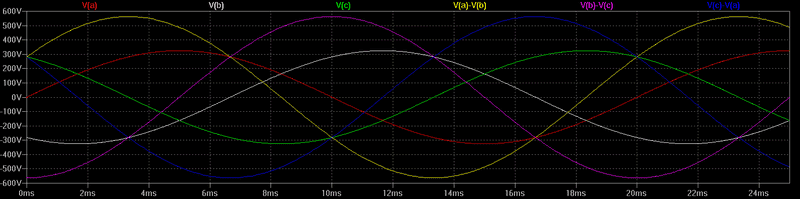# What exactly is the potential difference between phases in 3 phase power distribution?

oscarrod5
I understand phase voltage (phase to neutral) well, but I'm still confused by what exactly the potential difference is between any 2 phases in 3 phase power. If you were to try to find the potential difference where 2 sine wave phases cross, then at that instantaneous point, the potential difference looks to be 0.

https://en.wikipedia.org/wiki/Mathe...ric_power#/media/File:3_phase_AC_waveform.svg

Staff Emeritus
I think it is easiest to visualize using a vector diagram. Does the following help?Line-to-neutral voltages are in blue and line-to-line voltages in red. Note that the magnitude of the line-to-line is ##\sqrt{3}## times larger, and ±30 degrees different in phase compared to line-to-neutral.

••Klystron, DaveE, jim mcnamara and 2 others
Mentor
at that instantaneous point, the potential difference looks to be 0.

Yes. Have you taken into account it actually is not different from the case of a single phase and neutral: you can also find the instantaneous point at which potential difference is zero?

•Interested_observer
Gold Member
what exactly the potential difference is between any 2 phases in 3 phase power.
Here in the U.S. the 3-phase to residential areas is often 16kV between phases (DELTA connected). One phase of this is then stepped down to the usual 240V centertapped for residential use.

For larger apartment buildings with an elevator and air conditioning (and for larger restaurants) the 16kV 3-phase is stepped down to 120/208 3-phase/4-wire (WYE connected). This causes some complaints about electric stoves not heating as fast as they 'should.' Of course this is because of the 208V feeding a 240V stove. Try explaining that to an upset housewife!

Medium size industrial plants are often fed with 416/240 3-phase/4-wire with a 240/120V transformer on the customer site. This has its own quirk in that the transformer often has a fuse mounted right on the transformer, which is behind a blank panel on the circuit breaker box. If the maintenance guy hasn't run across this before, the lights may be out for quite a while during troubleshooting.There is/was a nominal 440V feed and a 480V feed, but I have no details on those.

Cheers,
Tom

Single phase, 230 Vrms, is now the international standard domestic voltage.
The peak single phase voltage is 230 * √2 = 325 Vpk.
See the plot of the 'Y', single phase voltagess a, b, and c, relative to the neutral.

The Δ difference voltages between phases are also shown. V(a)-V(b), V(b)-V(c), V(c)-V(a).
The difference voltages are 400 Vrms. The peak difference voltage is 400 * √2 = 563 Vpk.

The three phase voltage is root 3 times the single phase voltage.
230 Vrms * √3 = 400 Vrms.
325 Vpk * √3 = 563 Vpk.•Klystron
Gold Member
There is/was a nominal 440V feed and a 480V feed, but I have no details on those.
277/480 Wye is quite common in US industrial settings. My last company sold Lasers that used this feed and it was seldom a problem in any sort of place that would want a big laser. Everyone overseas had to buy an autotransformer though. The cooling water, OTOH, was always a PITA for everyone.

480/277 is the US Industrial Standard - I only remember seeing 440 in OLD scott connected arrangements in Philly.

While the vector diagram are helpful - I think they are best as the second or final step to looking at this. The 3 Phase waveform is the good first step - a vertical line on this diagram shoes the REAL TIME potential difference for each connection.

Gold Member
Homework Helper
I understand phase voltage (phase to neutral) well, but I'm still confused by what exactly the potential difference is between any 2 phases in 3 phase power. If you were to try to find the potential difference where 2 sine wave phases cross, then at that instantaneous point, the potential difference looks to be 0.

https://en.wikipedia.org/wiki/Mathe...ric_power#/media/File:3_phase_AC_waveform.svg
You can use the standard Mathematics expression to find the Potential Difference. notably, the difference between the two Sine functions. Sin A - Sin B = 2 x Cos [(A+B)/2] x Sin [(A-B)/2]
We used to recite that as " sine minus sine equals two cos a half sum, sine a half difference." (With similar expressions for sin plus sin, cos plus cos and cos minus cos)*

The phases in -phase electricity are 120 degrees difference, so you are looking at two expressions like Sin(A) and Sin(A+120). (if I could type pi I could do that in radians rather than degrees)
"a half sum" equals (A +60), but cos(A+60) is just a phase shifted Sin(A)
"a half difference" equals 120, but sin(120) = √3 / 2

so 2 x {cos half sum) x (sin half difference) effectively means √3 sin(A), so the 3-phase PD is √3 times the single phase PD.

*
sin plus sin equals two sine a half sum, cos a half difference
sin(A) + sin(B) = 2 . sin[(A+B)/2] . cos[(A-B)]/2
cos plus cos equals two cos a half sum cos a half difference
cos(A) + cos(B) = 2 . cos[(A+B)/2] , cos[(A-B)/2]
cos minus cos equals two sine a half sum sine a half difference reversed.
cos(A) - cos(B) = 2 . sin[(A+B)/2] . sin[(B-A)/2]